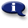# .

## Syllabus Information

Spring 2022
Dec 03,2023Use this page to maintain syllabus information, learning objectives, required materials, and technical requirements for the course.

Syllabus Information
MTH 065A - Elementary Algebra: Part A
Associated Term: Spring 2022
Learning Objectives: Upon completetion of this course, the student will: 1- Maintain, use, and expand skills and concepts learned in previous mathematics courses. Perform addition, subtraction, multiplication, and division of rational numbers. 2- Use and apply the concepts and language of algebraic expressions. 3- Solve linear equations and inequalities. Interpret information represented numerically and graphically, and recognize the linear relationships represented verbally, numerically, graphically, and algebraically. 4- Use information given verbally, numerically, or graphically about a linear relationship between two variables to write a linear equation which models the relationship. 5- Use the slope-intercept form or the point-slope form of the equation of a line to develop linear models. 6- Apply the concepts of linear modeling to a real-world situation of interest to the student. 7- Solve linear systems of two equations algebraically and graphically. 8- Make appropriate and efficient use of a scientific calculator. (Note: Students will be expected to demonstrate achievement of some objectives without the use of a calculator.)
Required Materials:
Technical Requirements: